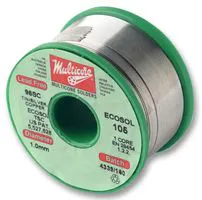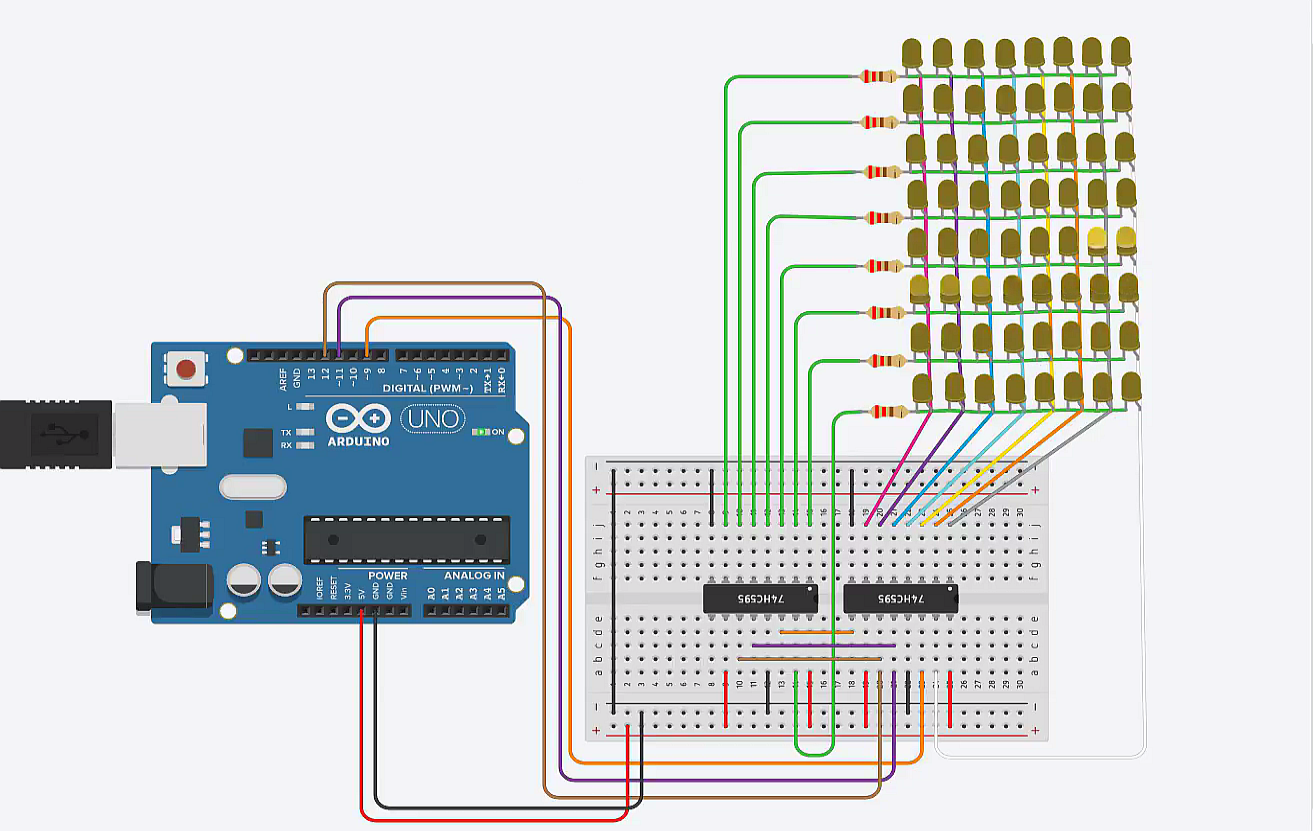Project tutorial

# Music Spectrum Visualizer with Arduino Nano © GPL3+

Visualizes the peak of frequencies with different bars on 8*8 led dot matrix using Arduino Nano.

• 4,474 views
• 11 respects

## Necessary tools and machinesSoldering iron (generic)## Apps and online services

The project is about how we can transform complex signal into simple or primary signals. Here we are using audio signal and make them decomposed into signals of different frequencies.

We are using Fourier transform, which helps us to decompose the signal. Fourier analysis converts a signal from its original domain (often time or space) to a representation in the frequency domain.

We are visualizing different frequency bands by their peak at the audio signal. And it can be seen on the 8*8 Led Dot Matrix.

Fourier Analysis has great computational cost, which can't be done on small computers. That's why we will be using Fast Fourier Transform, which is an algorithm that computes Discrete Fourier Transform of a sequence and has less computational cost, can be done on Arduino as well.

Arduino provides a library for Fast Fourier Transform which helps to get our work done more efficiently.

### How it works?

Here 3.5mm audio jack delivers signal from computer to the circuit. Then, it is fed to a capacitor rated 10 microfarad, which works as a filter. Then the connection is joined with Arduino analogue input A0, as we will be reading analogue value. Which is later joined with one resistor rated 1k ohms, is connected to the ground(0volts) and, another one, parallel to previous joint, is connected to positive(+5volts) for fixed gain.

### For Better Understanding:

How The Led Dot Matrix Works?

### Conclusion:

It was a fun experience working with the dot matrix and getting familiar with a new library, which was really exciting. We can also use LCD displays instead of led dot matrix. For large displays, all we need to change is to increase our sampling rate. At the end, It can be a great learning tool too!

Cheers!Happy Building.

## Code

##### Music VisualizerArduino
This is the main source code.
```#include "fix_fft.h"

#define CLOCK 3
#define LATCH 4
#define DATA 5

#define SAMPLES 32 //define according to your need

#define AUDIO A0

char im[SAMPLES];
char data[SAMPLES];
int barht[SAMPLES];

int binary[] = {1, 2, 4, 8, 16, 32, 64, 128};
int columnBinary[] = {1, 2, 4, 8, 16, 32, 64, 128};

void setup()
{
pinMode(CLOCK,OUTPUT);
pinMode(LATCH,OUTPUT);
pinMode(DATA,OUTPUT);
}

void loop()
{
static int i, j;
int val;

// get audio data
for(i = 0; i < SAMPLES; i++)
{
data[i] = (char)(val/4 - SAMPLES); // store as char
im[i] = 0; // init all as 0
}

// run FFT
fix_fft(data, im, 5, 0); //2^5=32, as we are taking 32 samples

// extract absolute value of data only, for 32 results
for(i = 0; i < SAMPLES/2; i++)
{
barht[i] = (int)sqrt(data[i] * data[i] + im[i] * im[i]);
}

for(i = 0, j = 0; i < SAMPLES/2; i++, j += 2)
{
barht[i] = barht[j] + barht[j + 1];
}

// display barchart
for(int k = 0; k < SAMPLES/4; k++){ //as we have 8rows and 8columns, so only 8 times we need to iterate. That's why 32/4=8 .
digitalWrite(LATCH, LOW);
int x = columnBinary[barht[k]%8]-1;
shiftOut(DATA, CLOCK, LSBFIRST, ~x); // columns
shiftOut(DATA, CLOCK, LSBFIRST, binary[k]); // rows
digitalWrite(LATCH, HIGH);
}
}
```
##### LED matrix test codeArduino
To test the custom built matrix
```byte scroll[]={
0B11111110,
0B11111101,
0B11111011,
0B11110111,
0B11101111,
0B11011111,
0B10111111,
0B01111111
};

byte point[]={
0B00000001,
0B00000010,
0B00000100,
0B00001000,
0B00010000,
0B00100000,
0B01000000,
0B10000000
};

int latchPin=11;
int clockPin=12;
int dataPin=9;

void setup() {
// put your setup code here, to run once:
pinMode(latchPin,OUTPUT);
pinMode(clockPin,OUTPUT);
pinMode(dataPin,OUTPUT);
}

void loop() {
// put your main code here, to run repeatedly:
for(int i=0;i<8;i++){
for(int j=0;j<8;j++){
digitalWrite(latchPin,LOW);
shiftOut(dataPin,clockPin,MSBFIRST,scroll[i]);
shiftOut(dataPin,clockPin,MSBFIRST,point[j]);
digitalWrite(latchPin,HIGH);
delay(100);
}
}
}
```
##### All codes
Find all the code and circuit layouts here.

## Schematics

Here the latch, data and clock pins are to be connected with the dot matrix, and we will be good to go.
cktdiagram_C7cnepXvi3.fzz
This schematic shows how to connect 64 LEDs with shift registers and arduino to make a LED dot matrix display work#### Sound Spectrum Visualizer with Arduino Nano 33 BLE

Project tutorial by Enrique Albertos

• 5,929 views
• 20 respects

#### Newbie Music Player

Project in progress by Michael Marinis

• 6,963 views
• 16 respects

#### Super-Simple Music Spectrum Visualizer

Project tutorial by Alex Wulff

• 8,638 views
• 9 respects

#### Out of My League - Music on Arduino

Project tutorial by MrZmann

• 2,533 views
• 4 respects

#### Music Reactive LED Strip

Project showcase by buzzandy

• 127,469 views